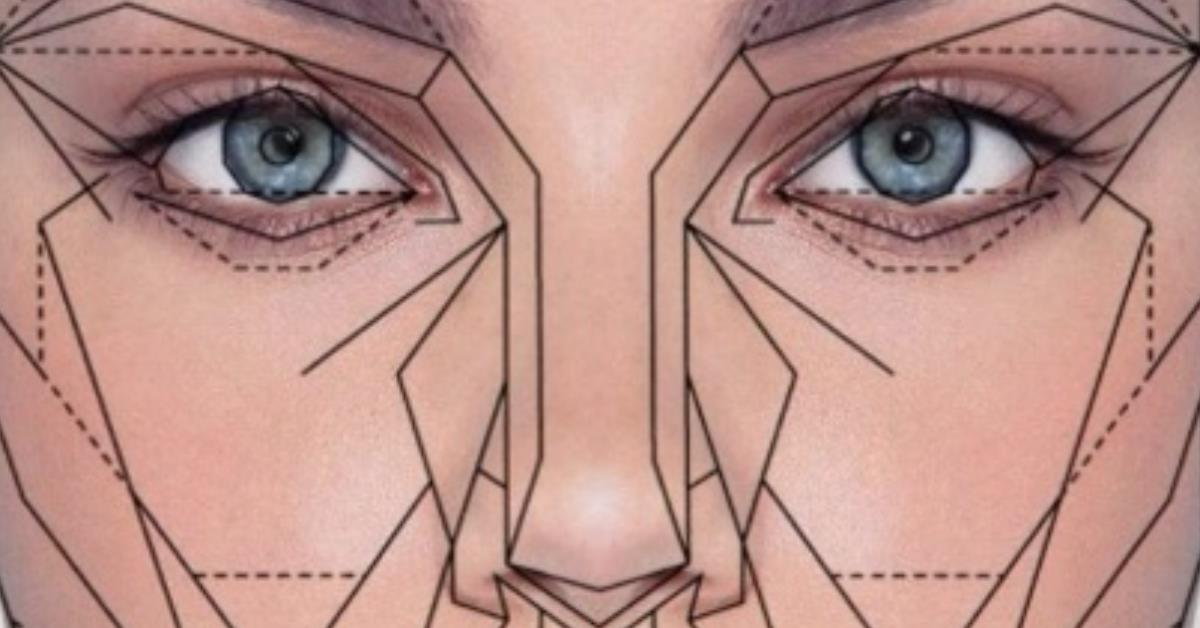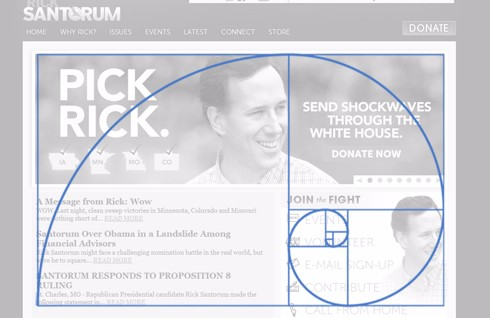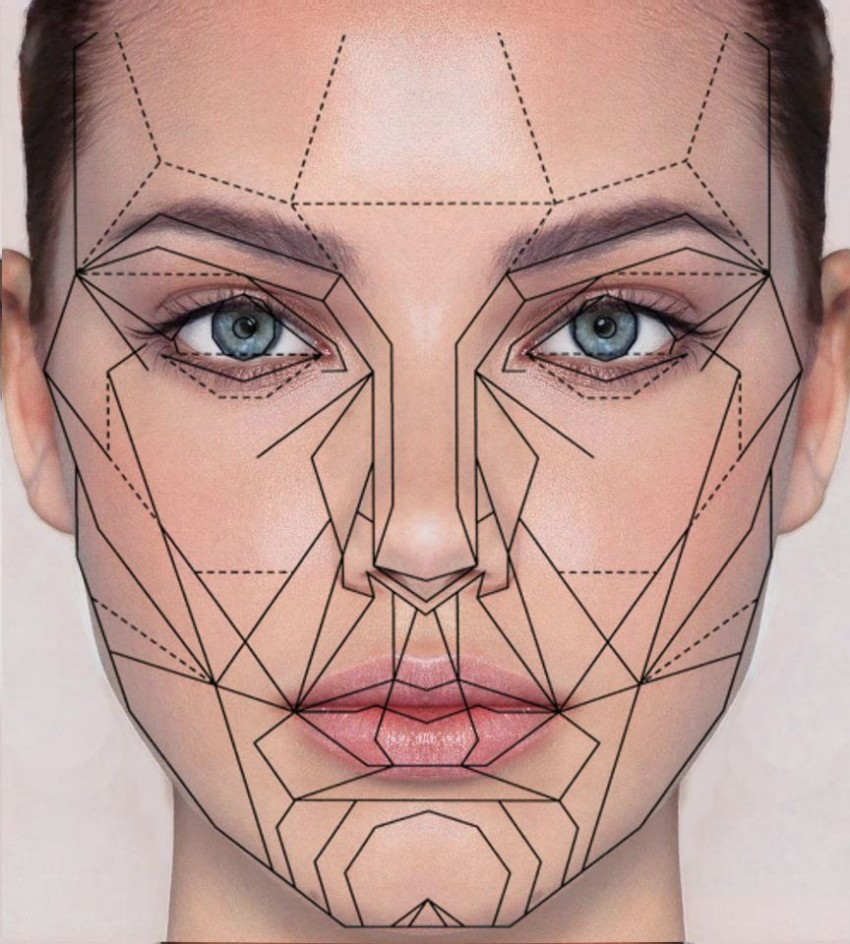### MagazineCertain limitations apply

----- magazine article -----

# Golden Ratio Calculator

What is it ? and How it works?

## What is Golden Ratio?

The golden ratio, also known as the golden section or golden mean, is a mathematical concept that describes the ratio of two quantities in a way that is considered pleasing to the eye in art and design. It is defined as the ratio of the larger quantity to the smaller amount, approximately equal to 1.6180339887. To calculate the golden ratio for a specific set of measurements or dimensions, you can use the formula: (a+b)/a = a/b = 1.6180339887. A and b are the two quantities in the golden ratio.

## Why do you want to use the golden ratio in your projects?

The main reason to bring the golden ratio methodology in any design object is simple - your final result will be much more visually attractive.

## Golden Ratio Calculator

The calculation of object sizes in your design project driven by the golden ratio methodology is next - you use 1.6 multiplier or proportion between two sites of the related object(s).

Below are a few Golden Ratio Examples.

 ↔side A ↔side B ↔A+B the sum of two sides

Golden ration calculation in this case:

A/B=(A+B)/A=1.618 = Golden Ratio

Three scenarios:

### 1) How can we find A and B when (A+B) is known?

A=(A+B)/1.618
B=A*0.618

Take for example
A+B=100 mm
then
A=100/1.618=61.80 mm
B=61.80 * 0.618= 38.192 mm

### 2) How can we find side A when side B is known?

A=B*1.618

Take for example
B=32 mm
then
A=32*1.618=51.78 mm

### 3) How can we find B when A is known?

B=A/1.618

Take for example
A=70 mm
then
B=70/1.618=43.26 mm

## Golden Ratio Daily Life. Examples.

### Golden Ration In Art### Golden Ration In Web Design

In web design, the golden ratio is often used to determine the ideal proportion of elements on a webpage, such as the layout's width and height, the columns' size, and the spacing between elements. The resulting design will be aesthetically pleasing and visually balanced by adhering to the golden ratio.

It's interesting to note that Golden Ratio can also be called Divine Proportion; Fibonacci numbers also relate to this ratio. The Fibonacci series(1,1,2,3,5,8,13,21,34,55...) has a mathematical connection with Golden Ratio.

It is not a must to use Golden Ratio to design the website; it's just a guide; the designer can choose the best proportion to use in their design and make it more visually appealing.### Golden Ration in Human BodyThx
@iamlucid
@joehowarddesign

## Golden Ratio. Math and Nature.

Thx
@vihartvihart Mathemusician and Virtual Reality Philosopher

Certain limitations apply

Keywords  #Golden Ratio Calculator  # USR9-117J34f5

 Talk to investor concierge how you may get the best-fit investment opportunities.Top Investment Opportunities

# Investment Categories